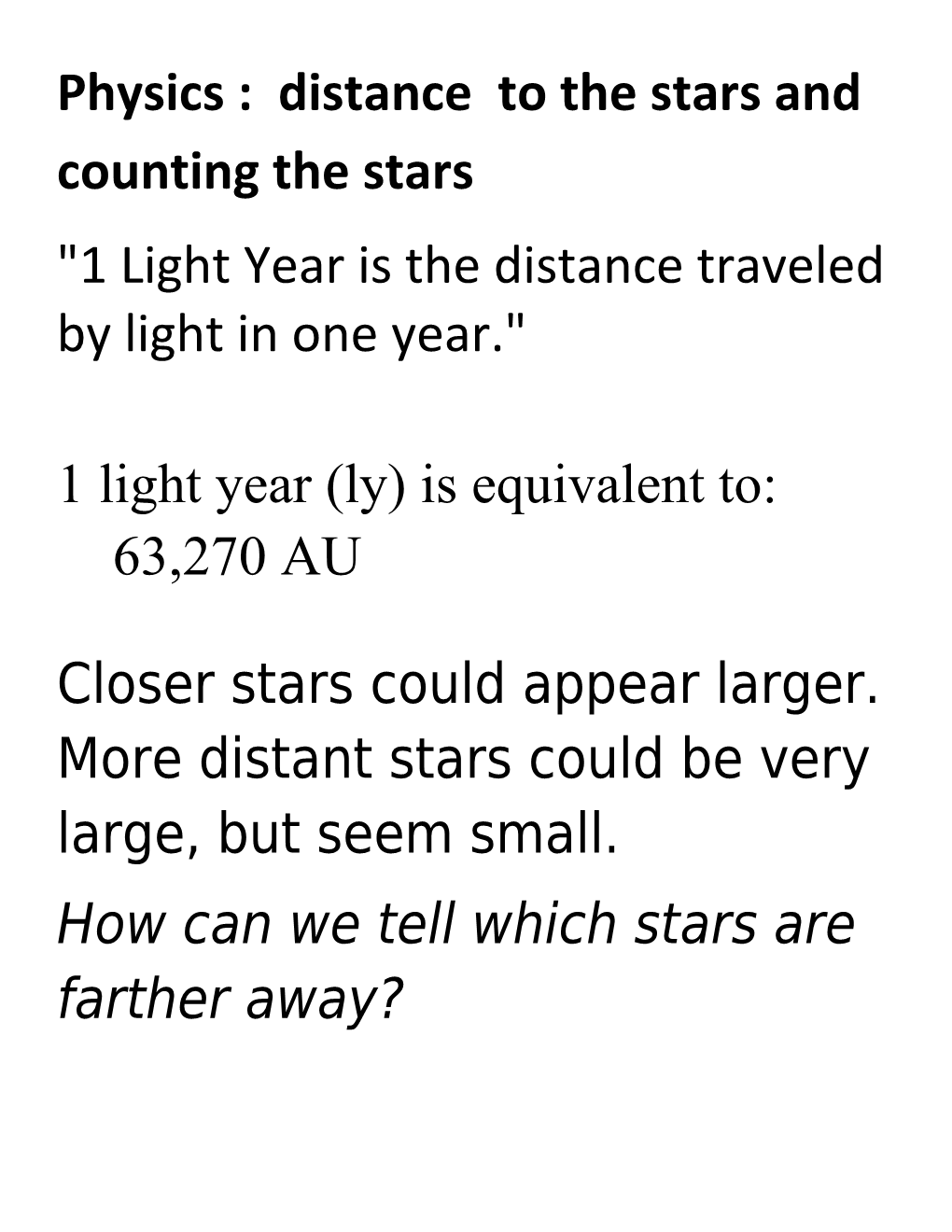# Physics : Distance to the Stars and Counting the StarsPhysics : distance to the stars and counting the stars

"1 Light Year is the distance traveled by light in one year."

1 light year (ly) is equivalent to:

63,270 AU

Closer stars could appear larger. More distant stars could be very large, but seem small.

How can we tell which stars are farther away?

Hold your hand out in front of you and look at it with your left eye closed, then your right eye closed. Your hand will appear to move against the background..

This is called parallax.As seen from the Earth, the nearby star appears to sweep through the angle shown.

Half of this angle, is the parallax, p.

Parallax Second = Parsec (pc)

Fundamental unit of distance in Astronomy

"A star with a parallax of 1 arcsecond has a distance of 1 Parsec."

A parsec is the fundamental unit of distance used by astronomers.

1 parsec =1 pc

1 pc= 3.3 ly = 3.09X1016 m

1 pc = 2.06X105 AU

Parallax decreases with distance

Calculating parallax:

Where:

p = parallax angle in arcseconds

d = distance in "Parsecs"

1 arcsecond = 1/3600 of 1 degree [there area 360 degrees in a circle]

60 arcsec = 1 arcminute= 1/60 of 1 degree

this formula works for stars that are up to 100 pc away.

Examples of Parallax Distances:

1. The star alpha Centauri has a parallax of p=0.742-arcsec:
1. A star is measured to have a parallax of p=0.02-arcsec:

HR diagrams - Categorizing Stars

Relationship between Luminosity and Mass

is the mass of the Sun

= 1.99 X10 30 kg

is the luminosity of the Sun

= 3.85X1026 Watts

Stars are on the main sequence if

Cooler Stars with very great mass are red giants or red supergiants

Hotter small stars are white dwarfs

[Mass of a star depends on its nebula size.]

Stars More Massive Than the Sun
1 solar mass has 1 solar luminosity (L)
2 solar mass stars have 10 L
4 solar mass stars have 100 L
8 solar mass stars have 1000 L
16 solar mass stars have 10000 L
32 solar mass stars have 100000 L
64 solar mass stars have 1000000 L / Stars Less Massive Than the Sun
1 solar mass has 1 L
1/2 solar mass stars have 1/10 L
1/4 solar mass stars have 1/100 L
1/8 solar mass stars have 1/1000 L
1/16 solar mass stars have 1/10000 L

Life sequence of a star depends on its mass

Medium stars eventually cool, expand into giants, lose mass, and then shrink into dwarfs

Many large stars explode as supernovas and then collapse into “neutrons stars”

Only very largest stars form black holes.

Life sequence of stars:You can also get black holes when dense objects, like neutron stars, collide with one another.

We believe there are the supermassive black holes at the heart of every galaxy. [Astronomers don’t know how those formed.]The radius of a black hole is called the “event horizon” or the Schwarzschild radius, rs.

Inside the event horizon, we see no light.

rs = 2MG

c2

where c = speed of light

G = universal gravitational constant

M = mass of star

Surrounding a black hole is a “photon sphere” which looks like a circle of light.

A photon sphere is a spherical region of space where gravity is strong enough so that photons are forced to travel in orbits.

The radius of the photon sphere is rp:

rp = 3MG = 3/2 rs

c2Question 1. What would happen to Earth if the Sun suddenly became a black hole?

[Earth would get very cold.

The gravitational force between Earth and the Sun would NOT change. WHY?]

Question 2: What would be the event horizon of the Sun if it could become a black hole?

Rs = 2MG/c2

= 2[1.99X1030 kg] [6.67X10-11 N m2/kg2 ]

[3X108 m/sec][3X108 m/sec]

rs = 2950 m =~ 3 km

question 3: What would be the photon radius of the Sun if it could became a black hole?

Rp = 3MG/c2= 3/2 Rs =~ 4.5 km

Rp = 3/2 Rs =~ 4.5 km

= 3[1.99X1030 kg] [6.67X10-11 N m2/kg2 ]

[3X108 m/sec][3X108 m/sec]

=~ 4500 m

= 3/2 Rs =~ 4.5 km

Question 4: What would be the radius of the Earth if it could become a black hole?

Rs = 2MG/c2

= 2[5.97X1024 kg] [6.67X10-11 N m2/kg2 ]

[3X108 m/sec][3X108 m/sec]

= .00885 m = 9 mm

How do we count the stars ?

Can we count them all?

Instead of counting every star, scientists estimate the number of stars using statistical sampling.

We count a small group and then ASSUME the rest of the

Black Hole Description

One of the terms used with black holes is "photon sphere", the radius of the orbit of light around the black hole. For 3 solar masses this radius is 13.5 km, 3/2 x the event horizon radius. The event horizon radius is also called the Schwarzschild radius.